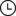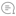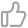# 【数学基础】从零构建统计随机变量生成器之离散基础篇机器学习初学者 |212020-11-24 06:04000

## 均匀分布（离散）

### 实现代码

import randomfrom math import floordef uniform(a: int, b: int) -> int:    assert a <= b    u = random.random()    return a + floor((b - a + 1) * u)

Github 代码地址：

https://github.com/MyEncyclopedia/stats_simulation/blob/main/distrib_sim/discrete_uniform.py

## 伯努利分布

### 实现代码

import randomdef bernoulli(p: float) -> int:    assert 0 <= p <= 1    u = random.random()    return 1 if u <= p else 0

Github 代码地址：

https://github.com/MyEncyclopedia/stats_simulation/blob/main/distrib_sim/discrete_bernoulli.py

## 类别分布

### 实现代码

import bisectimport randomfrom typing import Listdef categorical(probs: List[float]) -> int:    assert abs(sum(probs) - 1.0) < 0.001    cum = probs.copy()    for i in range(1, len(cum)):        cum[i] = cum[i-1] + probs[i]    u = random.random()    return bisect.bisect(cum, u)

Github 代码地址：https://github.com/MyEncyclopedia/stats_simulation/blob/main/distrib_sim/discrete_categorical.py

## 二项分布

### 实现代码

def binomial(n: int, p: float) -> int:    return sum(bernoulli(p) for _ in range(n))

Github 代码地址：

https://github.com/MyEncyclopedia/stats_simulation/blob/main/distrib_sim/discrete_binomial.py

## 几何分布

### 实现代码

from discrete_bernoulli import bernoullidef geometric(p: float) -> int:    fail_num = 0    while not bernoulli(p):        fail_num += 1    return fail_num

Github 代码地址：

https://github.com/MyEncyclopedia/stats_simulation/blob/main/distrib_sim/discrete_geometric.py

## 负二项分布

### 实现代码

from discrete_bernoulli import bernoullidef negative_binomial(r: int, p: float) -> int:    failures = 0    while r:        success = bernoulli(p)        if success:            r -= 1        else:            failures += 1    return failures

Github 代码地址：

https://github.com/MyEncyclopedia/stats_simulation/blob/main/distrib_sim/discrete_nagative_binomial.py

## 超几何分布

### 实现代码

from discrete_bernoulli import bernoullidef hypergeometric(N: int, K_succ_num: int, n_trial_num: int) -> int:    x = N - K_succ_num    n_hit = 0    while n_trial_num:        hit = bernoulli(K_succ_num / (K_succ_num + x))        n_hit += hit        if hit:            K_succ_num -= 1        else:            x -= 1        if K_succ_num == 0:            return n_hit        n_trial_num -= 1    return n_hit

Github 代码地址：

https://github.com/MyEncyclopedia/stats_simulation/blob/main/distrib_sim/discrete_hypergeometric.py

## 负超几何分布

### 实现代码

from discrete_bernoulli import bernoullidef negative_hypergeometric(N: int, K_success_num: int, r_fail_times: int) -> int:    fail_num = N - K_success_num    succ_trials = 0    while r_fail_times:        success = bernoulli(K_success_num / (K_success_num + fail_num))        if success:            K_success_num -= 1            succ_trials += 1            if K_success_num == 0: # no more success elements                return succ_trials        else:            fail_num -= 1            r_fail_times -= 1    return succ_trials

Github 代码地址：https://github.com/MyEncyclopedia/stats_simulation/blob/main/distrib_sim/discrete_negative_hypergeometric.py

## 伯努利试验总结往期精彩回顾适合初学者入门人工智能的路线及资料下载机器学习及深度学习笔记等资料打印机器学习在线手册深度学习笔记专辑《统计学习方法》的代码复现专辑AI基础下载机器学习的数学基础专辑获取本站知识星球优惠券，复制链接直接打开：https://t.zsxq.com/y7uvZF6本站qq群704220115。加入微信群请扫码：00

暂无评论~~
Ctrl+Enter